×#### Thank you for registering.

One of our academic counsellors will contact you within 1 working day.

Click to Chat

1800-1023-196

+91-120-4616500

CART 0

• 0

MY CART (5)

Use Coupon: CART20 and get 20% off on all online Study Material

ITEM
DETAILS
MRP
DISCOUNT
FINAL PRICE
Total Price: Rs.

There are no items in this cart.
Continue Shopping• Complete JEE Main/Advanced Course and Test Series
• OFFERED PRICE: Rs. 15,900
• View Details

```Chapter 16: Surface Area And Volume Exercise – 16.1

Question: 1

Solution:

Given that a solid sphere of radius = 8 cm

With this sphere, we have to make spherical balls = 1 cm

Since we don't know number of balls let us assume that number of balls be n

We know that,

Volume of the sphere = 4/3 πr3

The volume of the solid sphere equal to sum of the n spherical balls.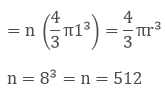Hence 512 numbers of balls can be made of radius 1 cm of a solid sphere of radius 8 cm.

Question: 2

How many spherical bullets each of 5 cm in diameter can be cast from a rectangular block of metal 11dm *1 m*5 dm?

Solution:

Given that a metallic block which is rectangular of dimension 11dm*1m*5dm

Given that the diameter of each bullet is 5 cm

Volume of the sphere = 4/3 πr3

Dimensions of the rectangular block = 11dm*1m*5 dm

Since we know that 1 dm = 10-1m

11*10-1*1*5*10-1 = 55*10-2 - ……..(i)

Diameter of the bullet = 5 cm

Radius of the bullet = 5/2 = 2.5 cm

So the volume of the rectangular block equals to the sum of the volumes of the n spherical bullets

Let the number of bullets be n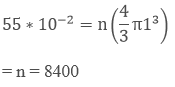Numbers of bullets formed were 8400.

Question: 3

A spherical ball of radius 3 cm is melted and recast into three spherical balls. The radii of the two balls are 2 cm and 1.5 cm respectively. Determine the diameter of the third ball?

Solution:

According to the question Radius of the spherical ball = 3 cm

We know that the volume of the sphere = 4/3 πr3

So it's volume (v) = 4/3 πr3

Given,

That the ball is melted and recast into 3 spherical balls.

Volume (V1) of first ball = 4/3 π 1.53

Volume (V2) of second ball = 4/3 π23

Radii of the third ball be = r cm

Volume of third ball (V3) = 4/3 πr3

Volume of the spherical ball is equal to the volume of the 3 small spherical balls.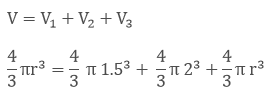Now,

Cancelling out the common part from both sides of the equation we get,

(3)3 = (2)3 + (1.5)3 + r3

r3 = 33- 23- 1.53 cm3

r3 = 15.6 cm3

r = (15.6)1/3 cm

r = 2.5 cm

We know diameter = 2* radius

= 2*2.5 cm

= 5.0 cm.

The diameter of the third ball is 5.0 cm

Question: 4

2.2 cubic dm of brass is to drawn into a cylindrical wire of 0.25 cm diameters. Find the length of the wire?

Solution:

Given,

2.2 dm3 of brass is to be drawn into a cylindrical wire of 0.25 cm

diameter Radius of the wire (r) = d/2

= 0.25/2 = 0.125*10-2 cm

Now, 1 cm = 0.01 m

So, 0.1cm = 0.001 m

Let the length of the wire be (h)

Volume of the cylinder = πr2h

Volume of brass of 2.2 dm3 is equal to volume of cylindrical wire.H = 448 m

The length of the cylindrical wire is 448 m

Question: 5

What length of a solid cylinder 2 cm in diameter be taken to recast into a hollow cylinder of length 16 cm, external diameter 20 cm and thickness 2.5 mm?

Solution:

According to the question

Diameter of the solid cylinder = 2 cm

The solid cylinder is recast into a hollow cylinder of length 16 cm, external diameter of 20 cm and thickness of 2.5 cm

Volume of the cylinder = πr2h

Radius of the cylinder = 1 cm

So, volume of the solid cylinder = π12h

Let the length of the solid cylinder be I

Volume of the hollow cylinder = πh(R2- r2).

Thickness of the cylinder = (R - r)

0.25 = 10 - r

Internal radius of the cylinder is 9.75 cm

Volume of the hollow cylinder = π × 16 (100 – 95.0625)

Hence, the volume of the solid cylinder is equal to the volume of the hollow cylinder

Equation I = equation ii

π12h = π × 16(100 - 95.06)

h = 79.04 cm

Length of the solid cylinder is 79.04 cm.

Question: 6

A cylindrical vessel having the diameter equal to its height is full of water which is poured into two identical cylindrical vessels with diameter 42 cm and height 21 cm which are filled completely. Find the diameter of the cylindrical vessel?

Solution:

Given,

The diameter is equal to the height of a cylinder = πr2h

So, volume = πr22r    (h = 2r)  ..... (i)

= 2πr2

Volume of each vessel = πr2h

Diameter = 42 cm

Height = 21 cm

Diameter = 2r

2r = 42 cm

r = 21 cm

Volume of vessel = π 212 × 21 ….. (ii)

Since the volumes of equation i and ii are equal

So equating both the equations

r3 = (21)3

r = 21 cm

d = 42 cm

The diameter of the cylindrical vessel is 42 cm.

Question: 7

50 circular plates each of diameter 14 cm and thickness 0.5 cm are placed one above the other to form right circular cylinder. Find its total surface area?

Solution:

Given that the 50 circular plates each with diameter 14 cm

Radius of circular plates = 7cm

Thickness of plates = 0.5 cm

Since these plates are one above the other so total thickness of plates = 0.5 *100 = 25 cm

Total surface area of a cylinder = 2πr × h + 2πr2

2πr (h + r)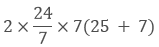Total surface area = 1408 cm2

The total surface area of the cylinder is 1408 cm2

Question: 8

25 circular plates each of radius 10.5 cm and thickness 1.6 cm are placed one above the other to form a solid circular cylinder. Find the curved surface area and volume of the cylinder so formed.

Solution:

Given that 250 circular plates each with radius 10.5 cm

Thickness is 1.6 cm.

Since plates are placed one above the other so its height becomes = 1.6*25 = 40 cm

Volume of the cylinder = πr2h

= π × 10.52 × 40 = 13860 cm3

Curved surface area of a cylinder = 2πrh

2π × 10.5 × 40 = 2640 cm2

Volume of the cylinder is 13860 cm3

Curved surface area of the cylinder is 2640 cm2

Question: 9

The diameter of a metallic sphere is equaled to 9 cm. It is melted and drawn into a long wire of diameter 2 mm having the uniform cross section. Find the length of the wire?

Solution:

Given,

Diameter of the metallic sphere = 9 cm

Radius of the metallic sphere = 9/2 cm = 4.5 cm

Volume of the sphere = 4/3 πr3

= 4/3 π × 4.53

= 381.703 cm3      ……   (i)

Diameter of the cylindrical wire = 2 mm = 2/10 cm = 0.2 cm

The radius of the cylindrical wire be = 0 2/2 cm = 0.1 cm

Let the height of the cylindrical wire be = h cm

Volume of the cylindrical wire = π × r2 × h

= π × 0.12 × h          ….. (ii)

Since the metallic sphere is melted and recast into a long cylindrical wire. So comparing and equating both the above-marked equations

We get,

381.703 = π × 0.12 × h   ….. (iii)

H = 12150 cm

The required length of the wire after recasting of the metallic sphere is 12150 cm.

Question: 10

Find the number of smaller sphere required if the radius of the larger ball is four times the radius of the smaller ball.

Solution:

According to the question,

Let the radius of the smaller ball be = r cm

Now, the radius of the larger ball be = 4r cm

Volume of the smaller sphere = 4π × r3    …… (i)

Volume of the larger sphere = 4/3 π × (4r)3   ….. (ii)

Now, dividing equation (ii) by (i). we get,

Number of smaller balls (n)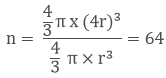The volume of the larger sphere is 8 times the volume of the smaller ball.

Curved surface area of the smaller sphere = 4 π r    ....(iii)

Curved surface area of the larger sphere = 4 π 2r4r .....(iv)

Now, dividing equation (iv) by (iii).

We get, Number of smaller spheres (N)The curved surface area of large ball is four times the curved surface area of the smaller ball.

Question: 11

A copper sphere of radius 3 cm is melted and re-casted into a right circular cone of height 3 cm. Find the radius of the base of the cone?

Solution:

Given, Radius of the sphere = 5 cm

Volume of the sphere = 4/3 π r3

= 4/3 π × 33 ….. (i)

The given sphere is melted and recast into a right circular cone

Height of the cone = 3 cm

Volume of the right circular cone = 1/3 π r2h

= 1/3 π × r2 × 3 ..... (ii)

Now comparing equation (i) and (ii) we get

r2 = 36

r = 6 cm

The radius of the cone is 6 cm.

Question: 12

A copper wire of diameter 1 cm and length 8 cm is drawn into a wire of length 18 m of uniform thickness. Find the thickness of the wire?

Solution:

Given, Diameter of the copper wire = 1 cm

Radius of the copper wire = 1/2 cm = 0.5 cm

Length of the copper rod = 8 cm

Volume of the cylinder = π r2h

= π × 0.52 × 10 ……. (i)

Length of the wire = 18 m = 1800 cm

Volume of the wire = π r2h

π r2 × 1800  ….. (ii)

Now equating both the equations. We get,

r2 = 200.00044

r = 0.033 cm

The radius or thickness of the wire is 0.033 cm

Question: 13

The diameters of the internal and external surface area of hollow spherical shell are 10cm and 6 cm respectively. If it is melted and recast into a solid cylinder of length of 24, find the diameter of the cylinder?

Solution:

Given, Internal diameter of the hollow sphere = 6 cm

Internal radius of the hollow sphere = 6/2 cm = 3 cm

External diameter of the hollow sphere = 10 cm

External radius of the hollow sphere = 10/2 cm = 5 cm

Volume of the hollow spherical shell = 4/3 π × R3 – r3

= 4/3 π × 53 – 33         …..  (i)

Given the length of the solid cylinder = 8/3 cm

Let the radius of the solid cylinder be h cm

Volume of the cylinder = π × r2 × h

= π × r2 × 8/3       ….. (ii)

Now equating both the above marked equations in order to obtain the radius of the cylinder

r2 = 49

r = 7

d =7*2 = 14 cm

Question: 14

How many coins 1.75 cm in diameter and 2 mm thick must be melted to form a cuboid 11 cm 10 cm 7 cm?

Solution:

So its volume = 11 × 10 × 10 cm3

Given diameter = 1.75 cm

Volume of the cylinder = πr2h

= π 0.8752 × 0.2

V1 = V2 × n

By calculating the above problem we get,

N = 1600

Number of coins are 1600

Question: 15

The surface area of the solid metallic sphere is 616 cm3. It is melted and recast into a cone of height 28 cm. find the diameter of the base of the cone so formed?

Solution:

The height of the cone = 28 cm

Surface area of the sphere = 616 cm3

We know that the surface area of the sphere = 4πr2

4πr2 = 616

r2 = 49

r = 7

Radius of the sphere = 7 cm

Let R be the radius of the cone

Volume of the cone = 1/3 πr2h

= 1/3 πr2 28      ..... (i)

Volume of the sphere = 4/3 πr3

= 4/3 π73  ..........  (ii)

Comparing equation (i) and (ii)

R2 = 49

R =7

Diameter of the cone = 7*2 = 14 cm

The diameter of the base of the cone is 14 cm

Question: 16

A spherical shell of internal and external diameter 6 cm and 10 cm respectively is melted and recast into a cylinder of diameter 14 cm. find the height of the cylinder ?

Solution:

Internal diameter of a hollow spherical shell = 6 cm

Internal radius of a hollow spherical shell = 3 cm

External diameter of a hollow spherical shell = 10 cm

External radius of a hollow spherical shell = 5 cm

Volume of the spherical shell = 4/3 π × (53 - 33)          … (i)

Diameter of the cylinder = 14 cm

Radius of the cylinder = 7cm

Let the height of the cylinder be (x) cm

Volume of the cylinder = π × 72 × x   ……… (ii)

According to the question;

Volume of the cylinder is equals to the volume of the spherical shell.

4/3 π × (53 - 33) = 49 × x

x = 2.666 cm

Question: 17

A hollow sphere of internal and external diameters 4cm and 8cm is melted into a cone of base diameter 8 cm.Calculate the height of the cone?

Solution:

Given, Internal diameter of hollow sphere = 4 cm

Internal radius of hollow sphere = 2 cm

External diameter of hollow sphere = 8 cm

External radius of hollow sphere = 4 cm

Volume of the hollow sphere 4/3 π × (43 - 23)          … (i)

Given,

diameter of the cone = 8 cm

Radius of the cone = 4 cm

Let the height of the cone be x cm

Volume of the cone 1/3 π × 42 × h          ….. (ii)

Since the volume of the hollow sphere and cone are equal. So, equating equations (i) and (ii)

we get,

4/3 π × (43 - 23) -  1/3 π × 42 × h

H = 12

The height of the cone so formed is having a height of 12 cm

Question: 18

A path 2 m wide surrounds a circular pond of diameter of 40 m. How many cubic meters of sand are required to grave the path to a depth of 20 cm?

Solution:

Given, Diameter of the circular pond = 40 m

Radius of the pond = 20 m/2 = 10 m (r = d/2)

Thickness = 2 m

We know 1 cm = 0.01 m

10 cm = 10/100 m = 0.10 m

Since the whole view of the pond looks like a hollow cylinder. So,

Thickness (t) = R – r

2 = R - 20

R = 22 m

Volume of the hollow cylinder = π (R2- r2) × h

= π (222- 202) × 0.10

= 52.77 m3

Volume of the cylinder is 52.77m3. Since it is a hollow cylinder the volume of the cylinder indicates the required amount of sand needed to spread across to a depth of 20 m.

Question: 19

A 16 m deep well with diameter 3.5 m is dug up and the earth from it is spread evenly to form a platform 27.5 m by 7m. Find the height of the platform?

Solution:

Let us assume the well is a solid right circular cylinder Radius(r) of the cylinder = 3.5/2 m = 1.75 m

Height or depth of the well (h) = 16 m

Volume of the cylinder = πr2h

= π × 1.752 × 16       …. (i)

Given,

The length of the platform (I) = 27.5 m

Breadth of the platform (b) =7 m

Let the height of the platform be x m

Volume of the rectangle = l*b*h

V = 25*5*x ..... ..... ..  (ii)

Since the well is spread evenly to form the platform

So equating equations (i) and (ii), we get

V1 = V2

π × 1.75 × 1.75 × 16 = 27.5 × 7 × x

x = 0.8 m

Therefore x = 80 cm

The height of the platform is 80 cm.

Question: 20

A well of diameter 2 m is dug 14 m deep. The earth taken out of it is evenly spread all around it to form an embankment of height 40 cm. find the width of the embankment?

Solution:

Radius of the circular cylinder (r) = 2/2 m = 1 m

Height of the well (h) = 14 m

Volume of the solid circular cylinder = π r2h

= π × 12× 14

Given,

The height of the embankment (h) = 40 cm = 0.4 m

Let the width of the embankment be (x) m.

Volume of the embankment = π × r2 × h

= π × (1 + x)2 – (1)2 × 0.4 ….. (ii)

Since the well is spread evenly to form embankment so their volumes will be same So equating equations i and ii, we get

π × r2 × h = π × (2.5 + x)2 – (2.5)2 – 0.20

x = 5 m

The width of the embankment is 5 m.

Question: 21

A well with inner radius 4 m is dug up and 14 m deep. Earth taken out of it has been evenly all around a width of 3 m it to form an embankment. Find the height of the embankment?

Solution:

Given;

Inner radius of the well = 4 m

Depth of the well = 14 m

Volume of the cylinder = π r2h

= π × 42 × 14 …. (i)

According to the question;

The earth taken out from the well is evenly spread all around it to form an embankment Width of the embankment = 3 m

Outer radii of the well = 3 + 4 m = 7 m

Volume of the hollow well = π (R2 – r2) × h

= π × (72 - 42) × h …… (ii)

Comparing both equations we get H = 6.78 m

The height of the well so formed is 6.78 m.

Question: 22

A well of diameter 3 m is dug up to 14 m deep. The earth taken out of it has been spread evenly all around it to a width of 3 m to form an embankment. Find the height of the embankment.

Solution:

Given Diameter of the well = 3 m

Radius of the well = 3/2 m = 1.5 m

Depth of the well = 14 m

Width of the embankment = 4 m

Radius of the outer surface of the embankment = 4 + 3/2 m = 5.5 m

Let the height of the embankment be = h m

Volume of the embankment = π (R2 – r2) × h

= π (5.52 – 1.52) × h            ….. (i)

Volume of earth dug out = π × r2 × h

= π × 22 × 14    ….. (ii)

Comparing both the equations we get; H = 9/8 m Height of the embankment is 9/8 m

Question: 23

Find the volume largest right circular cone that can be cut out of a cone of a cube whose edge is 9 cm.

Solution:

Given,

The side of the cube = 9 cm

The largest cone is curved from cube diameter of base of cone = side of the cube

2r = 9

r = 9/2 cm = 4.5 cm

Height of cone = side of cube

Height of cone (h) = 9 cm

Volume of the largest cone to fit in = 1/3 π × r2 × h

= 1/3 π × 4.52 × 9

= 190.92 cm3

The volume of the largest cone to fit in is having a volume of 190.92 cm3

Question: 24

Rain water which falls on a flat rectangular surface of length 6 m and breadth 4 m is transferred into a cylindrical vessel of internal radius 20 cm .What will be the height of water in the cylindrical vessel if a rainfall of 1 cm has fallen?

Solution:

Given,

Length of the rectangular surface = 6 m = 600 cm

Breadth of the rectangular surface = 4 m = 400 cm

Height of the perceived rain = 1 cm

Volume of the rectangular surface = length * breadth * height

= 600*400*1 cm3

= 240000 cm3  …………….. (i)

Given,

Radius of the cylindrical vessel = 20 cm

Let the height of the cylindrical vessel = h cm

Volume of the cylindrical vessel = π × r2 × h

= π × 202 × h         ….. (ii)

Since rains are transferred to cylindrical vessel

So, equating both the above marked equations:

240000 = π × 202 × h

H = 190.9 cm

The height of the cylindrical vessel is 190.9 cm.

Question: 25

A conical flask of water. The flask has base radius(r) and height (h). The water is poured into a cylindrical flask of base radius Mr. Find the height of water in the cylindrical flask?

Solution:

Given:

Height of the conical flask = h m

Volume of the cone = 1/3 π × r2 h           ….. (i)

Given;

Let the height of the flask be h1.

Volume of the cylinder = π × r2h

It's volume = 1/3 π × r2 × h ……  (ii)

Since water in conical flask is poured into cylindrical flask their volumes are same.

So, equations i and ii are same

1/3 π × r2 × h = 1/3 × π r2 × h

h1 = h/3m2

The required height of the cylindrical vessel is h/3m2.

Question: 26

A rectangular tank is 15 m long and 11m in breadth is required to receive entire liquid contents from a full cylindrical tank of internal diameter 21 m and length 5 m. Find the least height of the tank that will serve the purpose?

Solution:

Given,

Length of the rectangular tank = 15 m

Breadth of the rectangular tank = 11 m

Let the height of the rectangular tank be = h m

Volume of the rectangular tank = length *breadth' height

=15*11*h m3...... (i)

Given,

Radius of the cylindrical tank = 21/2 m = 10.5 m

Height of the tank = 5 m

Volume of the cylindrical tank = π × r2 × h

= π × 10.52 × 5 ……. (ii)

Since equation i and ii are having same volumes so, equating both equations We get,

165 × h = π × 10.52 × 5

h = 10.5 m

The height of the tank is 10.5 m.

Question: 27

A hemispherical bowl of internal radius 9 cm is full of liquid. This liquid is to be filled into cylindrical shaped small bottles each of diameter 3 cm and height 4 cm. How many bottles are necessary to empty the bowl?

Solution:

Given, The internal radius of the hemispherical bowl = 9 cm

Volume of the hemisphere = 4/3 π × r3

= 4/3 π × 93 …………… (i)

Given,

Diameter of the cylindrical bottle = 3 cm Radius = 3/2 cm = 1.5 cm

Volume of the cylinder = π × r2 × h

= π × 1.52 × 4    …..  (ii)

Volume of the hemispherical bowl is equals to (n) multiplied by volume of the cylindrical bottles. Now, Comparing equations i and ii, We get,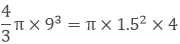N = 54

54 cylindrical bottles are required to empty a hemispherical bowl.

Question: 28

A cylindrical tube of radius 12 cm contains water to a depth of 20 cm. A spherical ball is dropped into the tube and the level of water is raised by 6.75 cm. Find the radius of the ball?

Solution:

Given, The radius of a cylindrical tube (r) = 12 cm

Level of water raised in the tube (h) = 6.75 cm

Volume of the cylinder = π × r2 × h

= π × 122 × h = π × 122 × 6.75

Let r be the radius of the spherical ball

Volume of the sphere = 4/3 π × r3 ……  (iii)

To find the radius of the spherical ball of unknown radius r we need to equate equations i and ii We get,

π × 122 × 6.75 = 12.571 × r3

r3 = 729

r = 9 cm

The radius of the spherical ball is 9 cm.

Question: 29

500 persons have to dip in a rectangular tank which is 80 m long and 50 m broad. What is the rise in the level of water in the tank, if the average displacement of water by a person is 0.04 m3?

Solution:

Given, The length of a rectangular tank (I) = 50 m

Breadth of the rectangular tank (b) = 10 m

Total displacement of water in a rectangular tank by 400 persons = 400* 0.02 m3

= 8 m3          ........  (i)

Let the depth of tank be h m

Volume of the rectangular tank = length * breadth * height

= I* b* h

= 50 × 10 × h m3 = 500 h m3 .. (ii)

Now equating equations i and we get,

8 = 500* h

H = 0.016 m

The rise in water level of the rectangular tank is 0.016 m.

Question: 30

A cylindrical jar of radius 6 cm contains oil. Iron spheres each of radius 1.5 cm are immersed in the oil. How many spheres are necessary to raise the level of the oil by 2 cm?

Solution:

Given,

The radius of the cylindrical jar (r) = 6 cm

Height of the cylindrical jar (h) = 2 cm

Let the number of balls be (n) Volume of the cylinder = π × r2 × h

= π × 62 × 2  …… (i)

Let the Radius of the sphere be 1.5 cm

Volume of the sphere = 4/3 π × r3   ….. (ii)

Volume of the cylindrical jar is equal to the sum of volume of (n) number of spheres.

Now, equating equations I and 0 we get,

π × 62 × 2  = n × 4.1904

N = 16

N = 16

16 spherical balls are required to raise the water level by 2 cm.

Question: 31

The metallic spheres each of radius 2 cm area packed into a rectangular box of internal dimension 16 cm* 8cm* 8cm where 16 spheres are packed the box is filled with preservation liquid. Find the volume of this liquid?

Solution:

Given; Radius of the metallic spheres = 2 cm

Volume of the sphere = 4/3 π r3

= 4/3 π 23 …… (i)

Total volume of the 16 spheres =  16 × 4/3 π 23

Volume of the rectangular box = 16 × 8 × 8 cm3  …..  (ii)

Subtracting equation (ii) from (i) we get the volume of the liquid

Volume of the liquid = (1024 - 536.16) cm3 = 488 cm3

The volume of the liquid is 488 cm3

Question: 32

A vessel in the shape of a cuboids' contains some water. If 3 identical spheres are immersed in the water, the level of water is increased by 2 cm. If the area of the base of the cuboids is 160 cm2 and its height is 12 cm. determine the radius of any of the spheres?

Solution:

Given,

The area of the cuboids'= 160 cm2

Level of water in the vessel increased = 2 cm

Volume of the vessel = 160 × 2 cm2 = 320 cm2 ......... (i)

Volume of each sphere = 4/3 π r3

Total volume of 3 spheres = 3 × 4/3 π r3  …… (ii)

Now equating equation (i) and (ii) we get

320 = 3 × 4/3 π r3

R = 2.94 cm

The radius of the sphere so obtained is 2.94 cm.

Question: 33

Water in a canal 1.5 m wide and 6 m deep is flowing with a sped of 10 km / hr .How much area will it irrigate in 30 minutes if 8 cm of standing water is desired?

Solution:

Given,

Water is flowing at a speed of = 10 km/hr

In 20 minutes length of the flowing standing water = 10 × 30/60 = 5 km = 5000 m

Width of the canal = 1.5 m

Depth of the canal = 6m

Volume of water in 20 minutes = 5000 × width × depth = 45000 m3

Irrigated area in 20 minutes if 5 cm of standing water is desired = 45000 / 0.08 m2 = 562500 m2

Irrigated area in 30 minutes is 562500 m2

Question: 34

A tent of height 77 dm is in the form of aright angled circular cylinder of diameter 36 m and height 44 dm surmounted by a right circular cone. Find the cost of canvas at the rate of Rs.3.5 per m2.

Solution:

Given The height of the tent = 77 dm

Height of the cone = 44 dm

Height of the tent without the cone = (77 - 44) dm = 33 dm = 3.3 m

Diameter of the cylinder = 36 m

Radius of the cylinder = 36/2 m = 18 m
Let us assume the slant height of the cone be (I)

L2 = r2 + h2

L2 = (18)2 + (3.3)2

I = 18.3 m

The slant height of the cone is 18.3 m.

Curved surface area of the cylinder = 2πrh

= 2 × π ×18 ×4.4 m2 ..... (i)

Curved surface area of the cone = πrh

= π × 18 × 18.3 m2  ……(ii)

Now adding equation (i) and (ii) we will get the value of the total surface area

Total surface area = 1532.46 m2

Cost of canvas = 1532.46 m2 × Rs 3.5 = Rs 5363.61

Question: 35

The largest sphere is to be curved out of a right circular cylinder of radius 7 cm and height 14 cm. find the volume of the sphere.

Solution:

Given radius of the cylinder = 7 cm

Height of the cylinder = 14 cm

Largest sphere is curved out from the cylinder.

Thus the diameter of sphere = diameter of cylinder Diameter of the sphere  = 2 × 7 = 14 cm

Volume of the sphere = 4/3 π r3

= 4/3 π 73

= 1436.75 cm3

Volume of the sphere is 1436.75 cm3

Question: 36

A right angled triangle whose sides are 3 cm, 4 cm and 5 cm is revolved about the sides containing the right angle in two ways. Find the difference in volumes of the two cones so formed. Also find the curved surfaces?

Solution:

Given:

Radius of the cone = 4 cm

Height of the cone = 3 cm

Slant height of the cone = 5 cm

Volume of the cone = 1/3 π r2 × h

= 1/3 × π 42 × 3

= 16 π cm3

Radius of the second cone = 3 cm

Height of the cone = 4 cm

Slant height of the cone = 5 cm

Volume of the cone = 1/3 π r2 × h

= 1/3 π 32 × 4

= 12 π cm3

Difference of the volumes of two cone = (16 - 12) π cm3

= 4 π cm3

Curved surface area of the first cone = πr1l1

= π × 4 × 5

= 20 π cm3

Curved surface area of the second cone = πr2l2

=π*3*5 cm2

= 15 π cm2

Question: 37

The volume of the hemisphere is 4851/2 find curved surface area?

Solution:

Given volume of the hemisphere = 2/3 π r3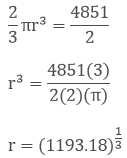r = 10.5 cm

Curved surface area of the hemisphere = 2πr2

= 2 × π × (10.5)2

The curved surface area of the hemisphere is 693 cm2

Question: 38

The difference between the outer and inner curved surface areas of a hollow right circular cylinder 14 cm long is 88 cm2. If the volume of metal used in making cylinder is 176 cm3. Find the outer and inner diameters of the cylinder?

Solution:

Given,

Height of the cylinder = 14 cm

Let the inner and outer radii of the hollow sphere be (r) and (R) respectively.

The difference between inner and outer curved surface area is 88 cm2

Curved surface area of the hollow sphere = 2π(R - r) h

88 = 2π(R - r) h

88 = 2π(R - r)× 14

R - r = 1     ..... (i)

Volume of the hollow cylinder = π × R2 – r2 × h

176 = π × R2 – r2 × h

176 = π × R2 – r2 × 15

From equation (i) substituting the values of equation. We get,

R + r = 4      ..... ..  (ii)

Now solving both the equations we get,

2R = 5

R = 2.5 cm & r = 1.5 cm

Inner radius of the hollow cylinder = 1.5 cm

Inner diameter of the hollow cylinder = 2 × 1.5 cm = 3 cm

Outer radius of the hollow cylinder = 2.5 cm

Outer diameter of the hollow cylinder = 5 cm

Question: 39

The internal and external diameters of a hollow hemispherical vessel are 21 cm and 25.2 cm .The cost of painting 1 cm2 of the surface is 10 paisa. Find the total cost to paint the vessel all over.

Solution:

Given,

Internal diameter of the hollow hemisphere = 21 cm

Internal radius of the hollow hemisphere = 21/2 = 10.5 cm

External diameter of the hollow hemisphere = 25.2 cm

External radius of the hollow hemisphere = 25.2/2 = 12.6 cm

Total area of the hollow hemisphere = 2πR2 + 2πr2 + π(R2 – r2)

= 2 π 12.62 + 2 π 10.52 + π (12.62 – 10.52)

= 997.51+ 692.72 + 152.39 cm2

= 1843.38 cm2

According to the question,

The cost of painting of 1 cm2 of the surface is = 10 p = Rs 0.01

Then the total cost of painting = 1848.38 × 0.10

= Rs 184.338

The total cost of painting all over the vessel is Rs.184.338.

Question: 40

The difference between outer and inner curved surface area of a hollow right circular cylinder is 14cm long is 88 cm2. If the volume of metal used in making cylinder is 176 cm3. Find the outer and inner diameters of the cylinder?

Solution:

Given,

Height of the hollow cylinder = 14 cm

Let the internal and external diameters of the hollow cylinder be r and R respectively.

Given that the difference between inner and outer curved surface = 88 cm3

Curved surface area of a hollow cylinder = 2 π (R - r) h

88 = 2π (R - r) × h

88 = 2 × 22/7 (R - r) × 14

R – r = 1  ……. (i)

Volume of the hollow cylinder = π (R2 – r2) × h

Volume of the hollow cylinder = 176 cm3

π(R2 - r2) h  = 176

R2 - r2  = 4

R + r =  4  ……  (ii)

Now, solving eq I and ii we get,

R - r = 1

R + r = 4

2R = 5

R =2.5 cm

r = 1.5 cm

The internal and external radii of the hollow cylinder are 1.5 cm and 2.5 cm respectively.

Question: 41

Prove that the surface area of a sphere is equal to the curved surface area of the circumscribed cylinder.

Solution:

Let the radius of the sphere be (r)

Curved surface area of the sphere = 4πr2   ........ (i)

S1 = 4πr2

Let the radius of the cylinder be (r)

Let the height of the cylinder be (2r)

Curved surface area of the cylinder = 2πrh

S2 = 2πr (2r)

= 4πr2    ........ (ii)

From the above equations, it is proven that surface area of the sphere is equal to the curved surface area of the circumscribed cylinder.

```### Course Features

• 728 Video Lectures
• Revision Notes
• Previous Year Papers
• Mind Map
• Study Planner
• NCERT Solutions
• Discussion Forum
• Test paper with Video Solution